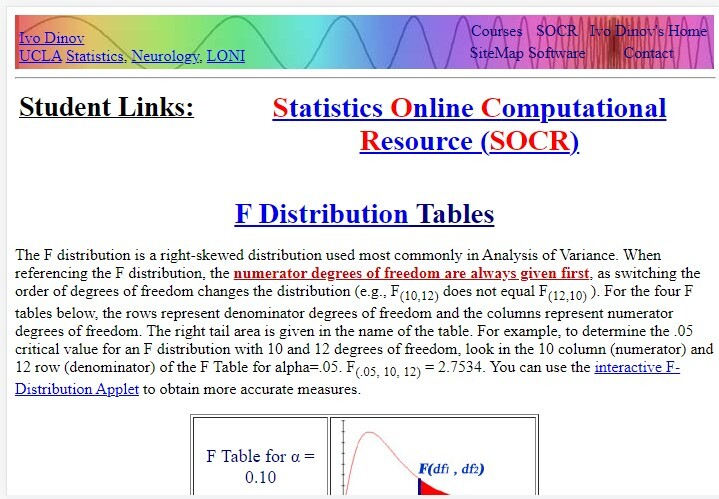# How to Perform an F-Test in Python

Statisticians use F-test to check whether the two datasets have the same variance or not. F-test is named after Sir Ronald Fisher. To use the F-Test, we make two hypotheses, a null hypothesis, and one alternate hypothesis. Then we select any of these two hypotheses approved by the F-Test.

Variance is a data distribution metric to tell the data deviation from the mean. The higher values show more dispersion than the smaller values.

In this article, you will learn how to perform an F-Test in Python programming Language with its use cases.

## F -Test Process

The process to perform the F-Test is as follows −

• To begin with, define the null and alternate hypotheses.

• Null Hypothesis or H0: σ12 = σ22 (the variances of the population are equal)

• Alternate Hypothesis or H1: σ12 ≠ σ22 (the variances of the populations are unequal)

• Choose the statistic for testing.

• Calculate the degrees of freedom for the populations. For instance, if m and n are population shapes, the degree of freedom is denoted as (df1) = m–1 and (df2) = n – 1 respectively.

• Now find the F value from the F-table.

• At last, divide the value of alpha by 2 for two-tailed tests to calculate the critical value.

Thus, we define the F value using the degrees of freedom of the populations. We read the df1 in the first row while df2 in the first column.

There are various F Tables for unique kinds of degrees of freedom. We compare the F statistic from step 2 with the critical value calculated in step 4. Then we can reject the null hypothesis if the critical value is lesser than the F statistic. On the contrary, we can accept the null hypothesis when the critical value is greater than the F statistic at some significant level.## Assumptions

We make some assumptions before performing the F-Test based on the dataset.

• The data population follows the normal distribution i.e. it fits the bell curve.

• Samples are non-correlated to each other i.e. no multicollinearity in the population.

Apart from these assumptions, we should also consider the following key points while performing the F-Test −

• The maximum variance value should be in the numerator to perform the right-tailed test.

• Determine the critical value after dividing alpha by 2 in the case of the two-tailed test.

• Check if you have variance or standard deviations.

• If you do not have degrees of freedom in the F Table, then go with the maximum value as the critical value.

## F-Test in Python

### Syntax

scipy stats.f()


### Parameters

x :  quantiles
q :  lower or upper tail probability
dfn, dfd shape parameters
loc :location parameter
scale :  scale parameter (default=1)
size :  random variate shape
moments : [‘mvsk’] letters, specifying which moments to compute


### Explanation

In this method, user must pass the f_value and iterable length of each array into the scipy.stats.f.cdf() and subtract it with 1 to perform the F-test.

### Algorithm

• First, import the NumPy and Scipy.stats libraries for the operation.

• Then create two lists of randomly chosen values with two different variable names and convert them into NumPy arrays and calculate the variance of each array using Numpy.

• Define a function to calculate the F-score where at first we divide the variances of arrays with a degree of freedom as 1.

• Then calculated the iterable length of each array and pass the f-value (ratio of variances), and the lengths into the CDF function, and subtracted this from 1 to calculate the p-value.

• Finally, p_value and f_value are returned by the function.

### Example

import numpy as np
import scipy.stats

# Create data
group1 = [0.28, 0.2, 0.26, 0.28, 0.5]
group2 = [0.2, 0.23, 0.26, 0.21, 0.23]

# Converting the list to an array
x = np.array(group1)
y = np.array(group2)

# Calculate the variance of each group
print(np.var(group1), np.var(group2))

def f_test(group1, group2):
f = np.var(group1, ddof=1)/np.var(group2, ddof=1)
nun = x.size-1
dun = y.size-1
p_value = 1-scipy.stats.f.cdf(f, nun, dun)
return f, p_value

# perform F-test
f_test(x, y)


### Output

Variances: 0.010464 0.00042400000000000017


You can observe that the F test value is 4.38712, and the respective p-value equals 0.019127.

We will abandon the null hypothesis since the p-value is less than .05. Thus, we can say that these two populations do not have equal variances.

## Conclusion

After reading this article, you now know how you can use F-Test to check if the two samples belong to the populations having the same variances. You have learned about the F-test process, assumptions, and Python implementation. Let us summarize the article with a few takeaways −

• The F test tells you if the two populations have equal variances.

• Calculate the degrees of freedom and calculate the critical value.

• Find the F-statistic from the F-Table and compare it with the crucial value calculated in the previous step.

• Accept or reject the null hypothesis based on the critical value and F-statistic comparison.

Updated on: 28-Apr-2023

2K+ Views